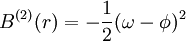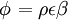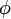# Martynov Sarkisov Vompe

Jump to: navigation, search

The Martynov-Sarkisov-Vompe (MSV) (Eq. 33 of ) closure relation is given in terms of the bridge function:$B^{(2)}(r) = - \frac{1}{2} (\omega - \phi)^2$

where$\left.\phi\right. = \rho \epsilon \beta$

where$\phi$ is short-ranged. The Weeks-Chandler-Andersen division of the Lennard-Jones potential was used. (Notice that the Martynov-Sarkisov-Vompe closure, for a hard sphere fluid, becomes the Martynov Sarkisov closure).